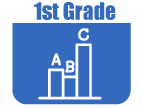IntroductionIn Grade 1, instructional time should focus on four critical areas: (1) developing understanding of addition, subtraction, and strategies for addition and subtraction within 20; (2) developing understanding of whole number relationships and place value, including grouping in tens and ones; (3) developing understanding of linear measurement and measuring lengths as iterating length units; and (4) reasoning about attributes of, and composing and decomposing geometric shapes.

(1) Students will develop strategies for adding and subtracting whole numbers based on their prior work with small numbers. They will use a variety of models, including discrete objects and length-based models (for example, cubes connected to form lengths), to model add-to, take-from, put-together, and take-apart; compare situations to develop meaning for the operations of addition and subtraction; and develop strategies to solve arithmetic problems with these operations. Students will understand connections between counting and addition and subtraction (for example, adding two is the same as counting on two). They will use properties of addition to add whole numbers and to create and use increasingly sophisticated strategies based on these properties (for example, "making tens") to solve addition and subtraction problems within 20. By comparing a variety of solution strategies, children will build their understanding of the relationship between addition and subtraction.

(2) Students will develop, discuss, and use efficient, accurate, and generalizable methods to add within 100 and subtract multiples of 10. They will compare whole numbers (at least to 100) to develop understanding of and solve problems involving their relative sizes. They will think of whole numbers between 10 and 100 in terms of tens and ones (especially recognizing the numbers 11 to 19 as composed of a ten and some ones). Through activities that build number sense, they will understand the order of the counting numbers and their relative magnitudes.

(3) Students will develop an understanding of the meaning and processes of measurement, including underlying concepts such as iterating (the mental activity of building up the length of an object with equal-sized units) and the transitivity principle for indirect measurement.

(4) Students will compose and decompose plane or solid figures (for example, put two triangles together to make a quadrilateral) and build understanding of part-whole relationships, as well as the properties of the original and composite shapes. As they combine shapes, they will recognize them from different perspectives and orientations, describe their geometric attributes, and determine how they are alike and different to develop the background for measurement and for initial understandings of properties such as congruence and symmetry.The Online Core Resource pages are a collaborative project between the Utah State Board of Education and the Utah Education Network. If you would like to recommend a high quality resource, contact Trish French (Elementary) or Lindsey Henderson (Secondary). If you find inaccuracies or broken links contact resources@uen.org.